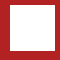Leon Anavi IT Tips && Tricks
 Navigation Home About Contact Disclaimer Categories All Administration C/C++ Databases Internet of Things Java Java ME Mobile & Embedded News PHP Reviews Tizen Web Mobile Applications location2sms Mobile Cipher### C/C++

Created: 31.08.2009 23:29 Last Modified: 31.08.2009 23:29 Views: 6055
Keywords: container, iterator, STL, vector

# STL Containers: Vector

## Purpose of the STL Vector

Vectors are STL (Standard Template Library) containers that support both direct and consecutive access to elements. Vectors support all the features of the native C++ arrays but have some advantages. Vectors are implemented as dynamic arrays so they can change their size automatically. The STL Vector is a template class so it supports multiple data types: all native C++ types and user defined types, structures and classes.

## Tricks with Vectors

### Add a New Element

• Add an element to the end of the vector
• The method push_back is used to add an element to the end of the container.
```#include <iostream>
#include <vector>
int main(int argc, char** argv)
{
std::vector<int> MyVector;
MyVector.push_back(1);
std::cout << MyVector << std::endl;
return 0;
}
```
• Add an element at certain position
• The method insert should be used to extend the vector by adding a new element at a certain position
```#include <iostream>
#include <vector>
int main(int argc, char** argv)
{
std::vector<int> MyVector;
//Add an element at the beginning with value 1
std::vector<int>::iterator Iter;
Iter = MyVector.begin();
MyVector.insert(Iter,1);
std::cout << MyVector << std::endl;
return 0;
}
```

### Delete an Element

• Delete an element at the end of the vector
• The method pop_back should be used to remove an element at the end of the vector.
```#include <iostream>
#include <vector>
int main(int argc, char** argv)
{
std::vector<int> MyVector;
MyVector.push_back(1);
MyVector.pop_back();
std::cout << MyVector.empty() << std::endl;
return 0;
}
```
The example add an element and after that deletes it. The execution of the application will result in 1 (true) because the vector will be empty.
• Delete an element at a certain position
• The method erase should be used to remove a certain element.
```#include <iostream>
#include <vector>
int main(int argc, char** argv)
{
std::vector<int> MyVector;
MyVector.push_back(11);
MyVector.push_back(21);
std::vector<int>::iterator Iter;
//Delete the first element
Iter = MyVector.begin();
MyVector.erase( Iter );
//Show the first element (aka the second before calling erase)
Iter = MyVector.begin();
std::cout << *Iter << std::endl;
return 0;
}
```
• Delete All Elements
• To truncate the vector call clear.
```#include <iostream>
#include <vector>
int main(int argc, char** argv)
{
std::vector<int> MyVector;
MyVector.push_back(11);
MyVector.push_back(21);
//Remove all elements
MyVector.clear();
std::cout << MyVector.empty() << std::endl;
return 0;
}
```

### Access to Elements of a STL Vector

• Loop through Elements with an Iterator
• ```#include <iostream>
#include <vector>
typedef std::vector<int> VectorInt;
typedef VectorInt::iterator VectorIntIter;
int main(int argc, char** argv)
{
VectorInt MyVector;
MyVector.push_back(11);
MyVector.push_back(21);
MyVector.push_back(31);
for(VectorIntIter Iter = MyVector.begin(); Iter < MyVector.end(); Iter++)
{
std::cout << *Iter << std::endl;
}
return 0;
}
```
• Direct Element Access
• ```#include <iostream>
#include <vector>
typedef std::vector<int> VectorInt;
int main(int argc, char** argv)
{
VectorInt MyVector;
MyVector.push_back(11);
MyVector.push_back(21);
MyVector.push_back(31);
int nSize = MyVector.size();
for(int nIter=0; nIter < nSize; nIter++)
{
std::cout << MyVector[nIter] << std::endl;
}
return 0;
}
```

# Class Reference

C++ : Reference : STL Containers : vector
C++ Vectors

 Home | About | Contact | Disclaimer | Sitemap © 2009-2018 Leon Anavi. All rights reserved.